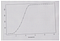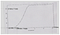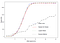# Introduction

My earlier post on wind turbine power curve fitting with a Weibull Cumulative Distribution Function (discussed here) is an excellent application of WindCurves, an R package. Here is another application of WindCurves package, i.e., to extract data from images or figures.

One of the advantages of the package is that it allows using the graph obtained by the manufacturer’s data as an input, while the output is the continuous function that fits that curve. With this package, R users will have a software tool that makes it easier for the use of power curves. The only thing a researcher will need is to have a set of pairs of points (wind speed, generated power), and the package will do its work and will provide a continuous function, based either on a logistic approximation or a Weibull cumulative distribution function (CDF).

It provides a feature to extract/capture wind speed versus generated wind power discrete points from power curve images. It works on a similar principle as the ‘digitize’ package, but in updated form, more precisely for wind power curves along with a few additional features.

# Extracting Discrete wind turbine (WT) Power Curve Points from a Power Curve Image

Generally, WT manufacturers provide the power curves in the form of graphs, and a manual ruler-based approach has been used to extract the wind speed and corresponding power values from the curve. A similar approach is exercised while replicating the power curves from research articles, books, or digital manuals. In order to minimize these efforts, ‘WindCurves’ provides the ‘img2points()’ function, which eases the process of extracting discrete WT power curve points from a power curve image. The ‘img2points()’ function works on a similar principle of the ‘digitize’ package but designed more specifically for WT power curves fitting with additional features. The ‘img2points()’ function has the following arguments:

imagePath: A character string for the path of an image of a power curve from which discrete values have to be extracted. This path can be absolute or relative within the working directory for the current R session. The ‘img2points()’ function uses this path and allows the user to select the desired points on the curve image.

n: An integer that represents the number of points to be captured on the curve image. The default value of the argument is fixed to 15.

The procedure to use the ‘img2points()’ function is as follows:

This function will import the desired image in the viewer panel and ask the user to locate two points on the X-axis and Y-axis of each of power curve image. Furthermore, it will ask the user to provide the actual values of the located four points on the image. The selection of any two points on each axis will work, but it is desired to select the extreme endpoints (with known values) of the curve image.

With these values, the ‘img2points()’ function maps the curve image and asks the user to point to ‘n’ points on the mapped image. Eventually, this function returns a data frame with two columns, wind speed, and generated power, that can be directly processed with the ‘fitcurve()’ function.

The ‘img2points()’ function operates similarly and as accurately as the ‘digitize’ package. The performance of the ‘digitize’ package gets deflected for the inclined input images. For such an inclined image, the points extracted from the curve are found to be deflected from its expected positions. The ‘img2points()’ function tackles such situations internally and retains the image with zero inclination in the X- and Y-axes. Eventually, this provides higher accuracy in extracting the discrete power curve points. Furthermore, in several cases, when the image is highly inclined and after relocating it to zero inclination of axes, the initial mapping of extreme ends of axes varies slightly and may produce a minute error in the system. Hence, in such cases, the ‘img2points()’ function asks the user to make the decision whether to map the extreme ends of axes again. The user can observe the deviation in mapping and accordingly decide whether to map the axes’ points again.

# Demonstration of ‘WindCurves’

This example demonstrates how ‘WindCurves’ can be used as a useful tool for analyzing and fitting the WT power curves. The objective of this demonstration is to become aware of the procedures to be followed when using the ‘WindCurves’ package to fit the WT power curves. In an earlier section, the ‘Nordex N90’ dataset was fitted, whereas in this section, the discrete values of the power curve were extracted from the curve image shown in the following figure.The WT power curve image to be supplied to the ‘img2points()’ function.

## Step 1: Extract Discrete Values of the Power Curve

This step involves two actions:

1. Select two extreme points on each X- and Y-axes (the following figure).

In this state, the inclination angle of the image (if there is one) is informed to the user who is asked to decide whether to re-map the X- and Y-axes’ points in the image. Furthermore, it transforms the inclined image with a new and non-inclined image. If the user permits the character “Y”, the ‘img2points()’ function will ask again to map the axes’ points, and more accurate mapping is achieved. Otherwise, the following action is activated.Selection and mapping of two points on each X- and Y-axes.

2. Point to ’n’ numbers of points on the power curve image. (the following figure)

## Step 2: Fit the Curve for Discrete Values Extracted in Step 1 with the ‘fitcurve()’ Function

The default syntax for the ‘fitcurve()’ function is as follows.

In this example, the following sample curve fitting method ‘random()’ is added in the study.

## Step 3: Compare the Fitted Curves

The default syntax for the ‘validate.curve()’ function is as follows.

In this example, the following addition sample error metric ‘error()’ (which returns the RSME values) is added in the study, and corresponding fitted curves can be plotted as shown below:The plot of the WT power curve fitted with the ‘fitcurve()’ function.

## Concluding remarks

The R package ‘WindCurves’ aims to enhance and speed up the research activities in the field of wind energy. The proposed package is quick and requires very little effort while working on WT power curves. This preliminary form of the package has included the Weibull CDF and logistic function methods, showing very promising results. Furthermore, it provides the provision to compare user-defined methods.

Author:

Dr. Neeraj Dhanraj Bokde,

Postdoctoral Researcher,

Aarhus University, Denmark

https://www.researchgate.net/profile/Neeraj_Bokde

Researcher in Data Sciences

## More from Neeraj Dhanraj

Researcher in Data Sciences